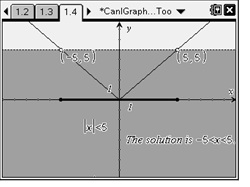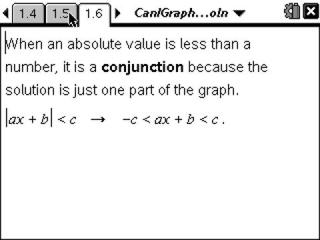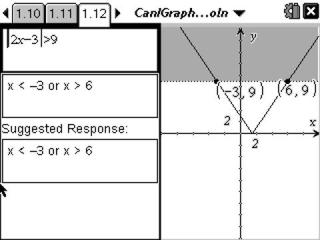# Activities

••• ##### Subject Area

• Math: Algebra I: Absolute Value Functions
• Math: Algebra I: Equations and Inequalities
• Math: College Algebra: Equations and Inequalities

• ##### Author9-12

15 Minutes

• ##### Device
• TI-Nspire™
• TI-Nspire™ CAS
• ##### Software

TI-Nspire™
TI-Nspire™ CAS

2.0

## Can I Graph You, Too?#### Activity Overview

In this activity, students will explore absolute value inequalities graphically, numerically, and algebraically. They will rewrite absolute value inequalities as compound inequalities without absolute value, then solve them.

#### Key Steps

•This activity is intended to be an introductory exploration into solving absolute value inequalities through graphing. The method of graphing is compared to the method used for finding the solution to an absolute value equation (graphing both sides and finding the intersection points).

•They are given the definition and graphical examples of disjunction and conjunction. A teacher demonstration may be required for students to fully understand the difference between the two terms.

•Students will first write the inequality as a conjunction or disjunction and then solve for x. They will then graph the left side and the right side using the Text tool and then find the intersection points. The dotted or solid lines will tell students if the intersection points are included or not included in the solution.